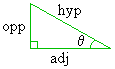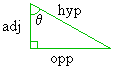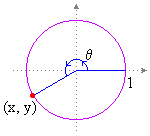Home    |    Teacher    |    Parents    |    Glossary    |    About UsEmail this page to a friendResources· Cool Tools · Formulas & Tables · References · Test Preparation · Study Tips · Wonders of MathSearchSine: Definition (Math | Algebra | Functions | Definition)

Sine: Definition

The sine function can be defined in a number of ways:

Definition I: From a triangleGiven any angle q (0 £ q £ 90°), we can find the sine of that angle by constructing a right triangle with one vertex of angle q. The sine is equal to the length of the side opposite to q, divided by the length of the triangle's hypotenuse. In this way, we can find the sine of any q in the range 0 £ q £ 90°.

Definition II: From the unit circleDraw a unit circle, in that a circle of radius 1, centered at the origin of a 2-dimensional coordinate system. Given an angle q, the sin(q) can be defined as the y-coordinate of the point on the circle that is located at an angle q from the origin. (According to standard convention, angles are measured counterclockwise from the positive horizontal axis.) In this way, we can find the sine of any real value of q (q Î Â).

Definition III: An algebraic approach

From defining a few general properties of the sine and cosine functions, we can algebraically derive the sine and cosine functions themselves.

Definition IV: Over the complex numbers

Given a complex number z = a + b i,
sin(z) = sin(a)cosh(b) + cos(a)sinh(b) i